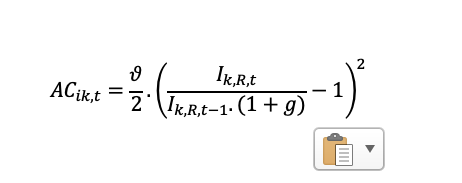# Investment adjustment costs in detrended form

Good morning everyone,If I detrend the model by growth (1+g), I get the following detrended adjustment cost function:But this function does not give me ac_ik=0 along the BGP. Hence, in order to have ac_ik=0 at SS, should I specify in the trend model that the adjustment cost function is the following:So that (1+g) goes away in the detrended model.

Thank you

There are two alternative, equivalent ways:
AC_{ik,t}=\frac{\vartheta}{2}\left(\frac{I_{k,R,t}}{I_{k,R,t-1}(1+g)}-1\right)^2
or
AC_{ik,t}=\frac{\theta}{2}\left(\frac{I_{k,R,t}}{I_{k,R,t-1}}-(1+g)\right)^2=\frac{\theta(1+g)^2}{2}\left(\frac{I_{k,R,t}}{I_{k,R,t-1}(1+g)}-1\right)^2
so that
\vartheta=\theta(1+g)^2

Thank you @jpfeifer.

I have fixed the adjustment cost parameter at 1.5. If I choose option number one, Does that mean that I should multiply that value by (1+g)^2 ?

Given that 1+g is usually small, it often does not matter. But the typical way is to have the first specification and treat \vartheta as the parameter from the detrended version of the model, i.e. keeping it at 1.5

1 Like